EQ Triangles Homepage

# On Breaking-down any Triangle intoa pair of Mirror-Imaged Equilateral Triangles

### Summary and Key Findings :

#### SUMMARY :

• This paper is an attempt to break-down any { 3-Points Shape } , such as a Triangle ,

• into a pair of mirror-imaged Equilateral Triangle , resized and/or re-oriented as the case may be .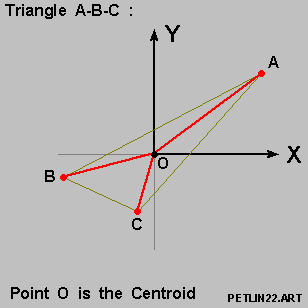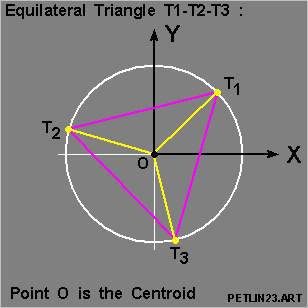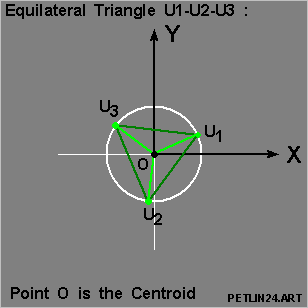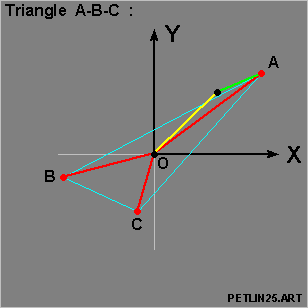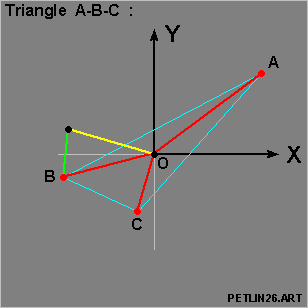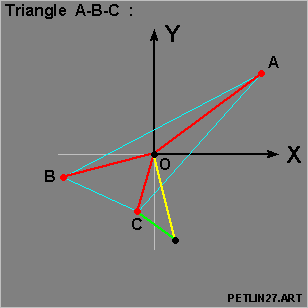• We then have the relations :

• [ Vector O-A ] = [ Vector O-T1 ] + [ Vector O-U1 ]

• [ Vector O-B ] = [ Vector O-T2 ] + [ Vector O-U2 ]

• [ Vector O-C ] = [ Vector O-T3 ] + [ Vector O-U3 ]

as per the set of 3 diagrams above .

In general , the centroid of the Triangle is used as the primary Point-of-Reference ,

• where as in the case of syzygy ( Point A/B/C lying on the same straight-line ) :

• the center-of-mass for a { 3-equal-mass system } of [ Mass A ] / [ Mass B ] / [ Mass C ]

• is used instead as the primary Point-of-Reference .

#### Key Finding One :

• We were successful in breaking-down any { 3-Points Shape } into its 2 mirror-imaged Equilateral Triangles .

This done via :

• First creating a random complex plane in the plane of the triangle , so that :

• the { REAL Axis } and the { IMAGINARY Axis } intersect at [ Point O ] , the centroid of { Triangle A-B-C } ;

• Secondly assign complex values to Point A / B / C based on their position on the Complex Plane ,

• Thirdly , asssume that the complex values A / B / C are the solution values to a { 3rd-Order Polynomial Equation } of the format :

•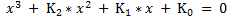and proceed to calculate the fixed values K2 / K1 / K0 based on the complex values A / B / C .

• Fourthly , solve the { 3rd-Order Polynomial Equation } via the Cardano Method ,

• and this will yield us the 2 Equilateral Triangles .

#### Key Finding Two :

• We were able to establish that the 3 Triangles , namely :

• the original { Triangle A-B-C } and its 2 broken-down Equilateral Triangles ,

are invariant upon Axis-Rotation and Distance Re-calibration ,

• in the solution procedure as detailed in { Key Finding One } above .

#### Key Finding Three :

• We identified an invariant-line associated with each-and-every triangle other than the Equilateral Triangle ,

• which we have named the { FENG-Line } for the time being to facilitate further discussions .

The { FENG-Line } also happens to be a Principal Axes of Rotation for a ( 3-equal-mass system } .

• The problem with the Equilateral Triangle here is two-folds :

• When we break-down an Equilateral Triangle into 2 component mirror-imaged Equilateral Triangles :

• one of the broken-down Equilateral Triangle is the same size as the original Equilateral Triangle ,

• whereas the other broken-down Equilateral Triangle is of size [ zero ] .

• The original Equilateral Triangle will have multiple Principal Axes of Rotation .

### Key Finding Four :

• We do observe here that many repetitive orbits for the { Equal-Mass Three-Body Problem } have been found .

We hope that :

• via breaking-down any { 3-Points Shape } into its 2 component mirror-imaged Equilateral Triangles ,

this will lead to a better understanding of the structural features and the stability of these orbits .

#### Table of Content

Part I --- Introduction and Overview Part II --- Setting up Reference System for the Triangles Section I Introduction Section II An Overview of the Paper Section III The Centroid as the Center of the Triangle Section IV Center-of-Mass for the Three-Body Problem with 3 Equal Masses Section V A First Look at Mirror-Imaged Triangles Section VI Outline and Purpose of Part III Section VII Constructing { Triangle A-B-C } Section VIII Some Operation Features of our Construction System Section IX Setting up the 3 Key Variables Section X Analysis for Region One Section XI Analysis for Region Two Section XII Analysis for Region Three Section XIII Analysis for Region Four Section XIV Analysis for Region Five Section XV Analysis for Region Six Section XVI Summarizing our Findings on the 6 Regions Section XVII Confirming the Existance of the 2 Equilateral Triangles Section XVIII Outline and Purpose for Part IV Section XIX Setting up the Random Triangle and the Reference Frame Section XX Creating the 3rd-Order Polynomial Equation from { Triangle A-B-C } Section XXI Solving the 3rd-Order Polynomial Equation Section XXII A Quick Review of the Multiplication of Complex Numbers Section XXIII Solving for [ T ] and [ U ] based on [ W1 ] Section XXIV Solving for [ T ] and [ U ] based on [ W2 ] Section XXV Summarizing Our Findings for Part IV Section XXVI Outline and Purpose for Part V Section XXVII Setting up the 2 different Reference Systems Section XXVIII Complex Values [ A ] / [ B ] / [ C ] - OLD vs. NEW Section XXIX 3rd-Order Polynomial Equation - OLD vs. NEW Section XXX [ W1 ] and [ W2 ] - OLD vs. NEW Section XXXI [ T1 ] / [ T2 ] / [ T3 ] - OLD vs. NEW Section XXXII [ U1 ] / U2 ] / [ U3 ] - OLD vs. NEW Section XXXIII Summarizing on Part V Section XXXIV Outline and Purpose for Part VI Section XXXV Setting up the Unit Circles - OLD vs. NEW Section XXXVI [ M ] and [ N ] - OLD System vs. NEW System Section XXXVII [ W1 ] and [ W2 ] - OLD System vs. NEW System Section XXXVIII [ T ] / [ U ] / [ X ] - OLD System vs. NEW System Section XXXIX Summarizing on Part VI Section XL Outline and Purpose for Part VII Section XLI Identifying the Invariant { FENG-Line } Section XLII Recalling the Random Triangle - { Triangle A-B-C } Section XLIII Recalling the 3rd-Order Polynomial Equation Section XLIV Solving the 3rd-Order Polynomial Equation again in Part VII Section XLV Setting up [ OMEGA-1 ] and [ OMEGA-2 ] Section XLVI Solving for [ Tau ] and [ Mu ] Section XLVII Explaining the { FENG-Line } and the { FENG-Circle } Section XLVIII The { FENG-Line } and { FENG-Circle } in action Section XLIX Principal Axes for a { 3-Equal-Mass System } Section L Confirming the { FENG-Line } as a Principal Axes Section LI Special Comment on the { Equal-Mass Three-Body Problem } Section LII Special Comment on { Mirror-Imaged Regular Pentagons } Section LIII Concluding Remarks